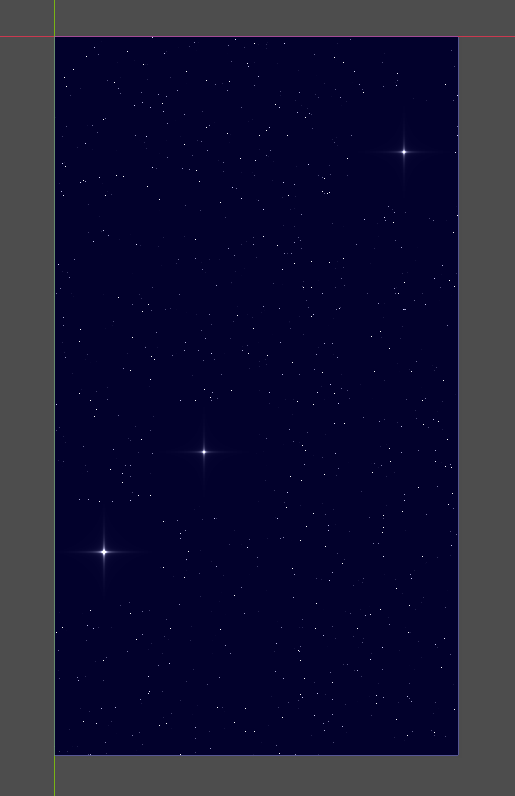Hi, if you like stars just like me then you can enjoy this shader. I played with an Android device and works really fine with a GLES2 project.

``````shader_type canvas_item;

uniform vec4 bg_color: hint_color;

float rand(vec2 st) {
return fract(sin(dot(st.xy, vec2(12.9898,78.233))) * 43758.5453123);
}

void fragment() {
float size = 100.0;
float prob = 0.9;
vec2 pos = floor(1.0 / size * FRAGCOORD.xy);
float color = 0.0;
float starValue = rand(pos);

if (starValue > prob)
{
vec2 center = size * pos + vec2(size, size) * 0.5;
float t = 0.9 + 0.2 * sin(TIME * 8.0 + (starValue - prob) / (1.0 - prob) * 45.0);
color = 1.0 - distance(FRAGCOORD.xy, center) / (0.5 * size);
color = color * t / (abs(FRAGCOORD.y - center.y)) * t / (abs(FRAGCOORD.x - center.x));
}
else if (rand(SCREEN_UV.xy / 20.0) > 0.996)
{
float r = rand(SCREEN_UV.xy);
color = r * (0.85 * sin(TIME * (r * 5.0) + 720.0 * r) + 0.95);
}
COLOR = vec4(vec3(color),1.0) + bg_color;
}``````
###### Tags
stars background motionThe shader code and all code snippets in this post are under CC0 license and can be used freely without the author's permission. Images and videos, and assets depicted in those, do not fall under this license. For more info, see our License terms.

## More from gerardogc2378

### Clouds in motion